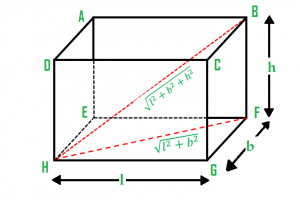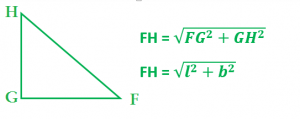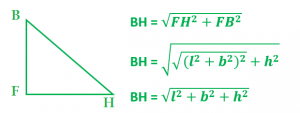# Length of longest rod that can fit into a cuboid

Given the length, breadth, and height of a cuboid, the task is to find the length of the longest rod that can fit in a cuboid.

Examples:

```Input: length = 12, breadth = 9, height = 8
Output: 17

Input: length = 22, breadth = 19, height = 8
Output: 30.1496
```

## Recommended: Please try your approach on {IDE} first, before moving on to the solution.Explanation: In the figure

`GH = length, GF = breadth, FB= height`

The length of the longest rod is BH. Therefore to compute BH we can apply Pythagoras Theorem in Triangle BHF. From triangle HGF we can compute the length of FH.After getting the length of FH we can find the length of BH using Pythagoras theorem in triangle BHF.Below is the implementation of the above approach:

## C++

 `// C++ program to find the longest rod ` `// that can fit in a cuboid ` `#include ` `using` `namespace` `std; ` ` `  `// Function to find the length ` `double` `longestRodInCuboid(``int` `length,  ` `                        ``int` `breadth, ``int` `height) ` `{ ` `    ``double` `result; ` `    ``int` `temp; ` ` `  `    ``// temporary variable to hold ` `    ``// the intermediate result ` `    ``temp = length * length + breadth * breadth  ` `           ``+ height * height; ` ` `  `    ``// length of longest rod is calculated ` `    ``// using square root function ` `    ``result = ``sqrt``(temp); ` ` `  `    ``return` `result; ` `} ` ` `  `// Driver code ` `int` `main() ` `{ ` `    ``int` `length = 12, breadth = 9, height = 8; ` ` `  `    ``// calling longestRodInCuboid() function to ` `    ``// get the length of longest rod ` `    ``cout << longestRodInCuboid(length, breadth, height); ` ` `  `    ``return` `0; ` `} `

## Java

 `// Java program to find the longest ` `// rod that can fit in a cuboid ` `class` `GFG  ` `{ ` ` `  `// Function to find the length ` `static` `double` `longestRodInCuboid(``int` `length,  ` `                                 ``int` `breadth,  ` `                                 ``int` `height) ` `{ ` `    ``double` `result; ` `    ``int` `temp; ` `     `  `    ``// temporary variable to hold ` `    ``// the intermediate result ` `    ``temp = length * length + breadth *  ` `           ``breadth + height * height; ` `     `  `    ``// length of longest rod is calculated ` `    ``// using square root function ` `    ``result = Math.sqrt(temp); ` `     `  `    ``return` `result; ` `} ` ` `  `// Driver Code ` `public` `static` `void` `main(String[] args)  ` `{ ` `    ``int` `length = ``12``,  ` `        ``breadth = ``9``, ` `        ``height = ``8``; ` ` `  `    ``// calling longestRodInCuboid()  ` `    ``// function to get the length  ` `    ``// of longest rod ` `    ``System.out.println((``int``)longestRodInCuboid(length, ` `                                    ``breadth, height)); ` `} ` `} ` ` `  `// This code is contributed by ChitraNayal `

## Python 3

 `# Python 3 program to find the longest rod  ` `# that can fit in a cuboid ` ` `  `# from math lib. import everything ` `from` `math ``import` `*` ` `  `# Function to find the length  ` `def` `longestRodInCuboid(length, breadth, height) : ` ` `  `    ``# temporary variable to hold  ` `    ``# the intermediate result ` `    ``temp ``=` `length ``*` `length ``+` `breadth  ``*` `breadth ``+` `height ``*` `height ` ` `  `    ``#  length of longest rod is calculated  ` `    ``# using square root function ` `    ``result ``=` `sqrt(temp) ` ` `  `    ``return` `result ` ` `  ` `  `# Driver Code ` `if` `__name__ ``=``=` `"__main__"` `: ` ` `  `    ``length, breadth, height ``=` `12``, ``9``, ``8` ` `  `    ``# calling longestRodInCuboid() function to  ` `    ``# get the length of longest rod ` `    ``print``(longestRodInCuboid(length, breadth, height)) ` ` `  ` `  `# This code is contributed by ANKITRAI1 `

## C#

 `// C# program to find the longest ` `// rod that can fit in a cuboid ` `using` `System; ` `class` `GFG  ` `{ ` ` `  `// Function to find the length ` `static` `double` `longestRodInCuboid(``int` `length,  ` `                                ``int` `breadth,  ` `                                ``int` `height) ` `{ ` `    ``double` `result; ` `    ``int` `temp; ` `     `  `    ``// temporary variable to hold ` `    ``// the intermediate result ` `    ``temp = length * length + breadth *  ` `        ``breadth + height * height; ` `     `  `    ``// length of longest rod is calculated ` `    ``// using square root function ` `    ``result = Math.Sqrt(temp); ` `     `  `    ``return` `result; ` `} ` ` `  `// Driver Code ` `public` `static` `void` `Main()  ` `{ ` `    ``int` `length = 12,  ` `        ``breadth = 9, ` `        ``height = 8; ` ` `  `    ``// calling longestRodInCuboid()  ` `    ``// function to get the length  ` `    ``// of longest rod ` `    ``Console.WriteLine((``int``)longestRodInCuboid(length, ` `                                    ``breadth, height)); ` `} ` `} ` ` `  `// This code is contributed by inder_verma.. `

## PHP

 ` `

Output:

```17
```

Attention reader! Don’t stop learning now. Get hold of all the important DSA concepts with the DSA Self Paced Course at a student-friendly price and become industry ready.

My Personal Notes arrow_drop_upCheck out this Author's contributed articles.

If you like GeeksforGeeks and would like to contribute, you can also write an article using contribute.geeksforgeeks.org or mail your article to contribute@geeksforgeeks.org. See your article appearing on the GeeksforGeeks main page and help other Geeks.

Please Improve this article if you find anything incorrect by clicking on the "Improve Article" button below.